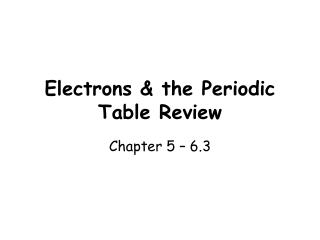Download PresentationElectrons & the Periodic Table Review

# Electrons & the Periodic Table Review - PowerPoint PPT Presentation

Electrons & the Periodic Table Review. Chapter 5 – 6.3. Given on the Test!!!!! c = 3.00 x 10 8 m/s h = 6.626 x 10 -34 Js c = f E = hf . Find the energy of an infrared photon whose frequency is 2.0  10 12 Hz. f = 2.0 x 10 12 1/s h = 6.626 x 10 -34 Js E = ? E = hfI am the owner, or an agent authorized to act on behalf of the owner, of the copyrighted work described.
Download Presentation## Electrons & the Periodic Table Review

Download Policy: Content on the Website is provided to you AS IS for your information and personal use and may not be sold / licensed / shared on other websites without getting consent from its author.While downloading, if for some reason you are not able to download a presentation, the publisher may have deleted the file from their server.

- - - - - - - - - - - - - - - - - - - - - - - - - - E N D - - - - - - - - - - - - - - - - - - - - - - - - - -
Presentation Transcript
1. Electrons & the Periodic Table Review Chapter 5 – 6.3

2. Given on the Test!!!!! c = 3.00 x 108 m/s h = 6.626 x 10-34 Js c = f E = hf

3. Find the energy of an infrared photon whose frequency is 2.0  1012 Hz. f = 2.0 x 1012 1/s h = 6.626 x 10-34 Js E = ? E = hf E = (6.626 x 10-34 Js)(2.0 x 1012 1/s) E = 1.3 x 10-21 J

4. 2. What is the wavelength of a red light wave whose frequency is 4.3  1014 Hz? c = f   = c/f 7.0 x 10-7 m 3. Calculate the frequency of an X-ray wave that has a wavelength of 9.2 nm.  = 9.2 x 10-9 m c = f  f = c/  3.3 x 1016 Hz

5. 4. What is the energy of a cosmic ray photon whose wavelength is 2.0 pm? • = 2.0 x 10-12 m 9.9 X 10-14 J 5. Find the frequency of a microwave that carries 3.4  10-25 J of energy. E = hf  f = E/h 5.1 x 108 Hz

6. photon 6. A packet of light energy that carries a quantum of energy. 7. The state when all electrons of an atom are in the lowest possible energy levels. 8. When an electron jumps up to a higher energy level, the atom is in its ___. 9. The scientist who applied Einstein’s particle-wave theory to electrons. 10. The theory that it is impossible to know both the position and speed of an electron simultaneously. 11. The theory that no two electrons in an atom can share the same 4 quantum numbers. ground state excited state Louis de Broglie Heisenberg uncertainty principle Pauli exclusion principle

7. Aufbau principle 12. The theory that electrons fill the lowest energy orbitals first. 13. The theory that, within a sublevel, electrons prefer to occupy their own orbital. 14. A term describing the outermost electrons in an atom. 15. A positively charged ion is called a(n) ______. A negatively charged ion is called a(n) _______. 16. The lower, left-hand section of the periodic table that includes most of the elements. 17. The upper right-hand section of the periodic table. Hund’s rule Valence electrons cation anion metals non-metals

8. Transition metals 18. The section of the periodic table that includes only the elements in the d-block. 19. The section of the periodic table that includes both the s-block and the p-block. 20. A three-dimensional region in space where an electron is likely to exist. 21. The process by which the core electrons partially block the attraction between the nucleus and the valence electrons. 22. What is the basis for exceptions to the aufbau diagram? Representative elements Energy level; sublevel; orbital shielding Full and ½ full sublevels are stable (i.e. Cr)

9. speed 33. According to the Heisenberg uncertainty principle, if the position of a moving particle is known, what other quantity CANNOT be known? 34. How many unpaired electrons are in a sulfur atom (Z = 16)? 35. The principal quantum number indicates what property of an electron? 36. What is the maximum number of electrons in the second principal energy level? 37.If three electrons are available to fill three empty 2p atomic orbitals, how will the electrons be distributed? 38. When an electron moves from a lower to a higher energy level, the electron _____. 2 Energy level 8 2n2 & n2 One electron in each orbital Absorbs energy

10. Lowest energy 39. According to the aufbau principle, electrons enter orbitals of ___ first. 40. What is the maximum number of “f” orbitals in any single energy level of an atom? 41. What is the charge of a cation? 42. Which of these elements has the lowest electronegativity value: a) cesium, or b) calcium? 43.What element in the second period has the largest atomic radius? 43. What is the element with the highest electronegativity value: a) calcium, or b) fluorine? 7 positive cesium Li - Lithium F - Fluorine

11. 44. Of the following, which one has the smallest first ionization energy: a) aluminum, or b) silicon? 45. Which of the following elements has the smallest ionic radius: a) Li, or b) K Al - Aluminum Li - Lithium